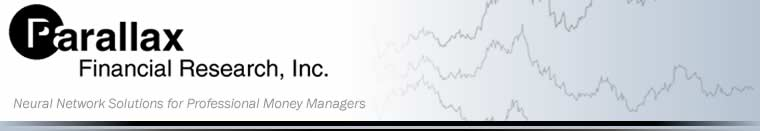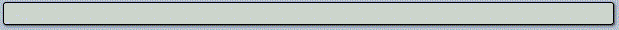Our Technology | Feedback | Scale Invariance | Spatial Probability | Neural Networks | Validation

## Neural Networks

A neural network is a mathematical modeling tool that has the capacity to learn by example. This is an extraordinarily useful ability, especially in financial modeling, where the predictive inputs are usually known and there are countless example forecasts. Networks are "trained" by entering hundreds of facts. Each fact consists of inputs and corresponding outputs. Through a unique feedback process, the network learns how those inputs are related to the outputs, and develops an internal model that describes the relationship.

Neural networks differ from standard analysis and are better suited to real world problems. Mathematical models are normally built by making a priori assumptions about the functional form of the solution. These are called parametric models, and are solved by regression methods to determine a number of coefficients. This is sufficient if the solution is known to be a second order polynomial, or some other simple, well-known function. But in the real world, functional relationships are not necessarily simple. Inputs and outputs often have a non-linear relationship. Neural networks offer a distinct advantage because the functional form of the answer is not required.